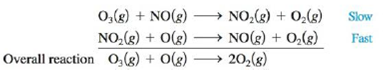# One mechanism for the destruction of ozone in the upper atmosphere is a. Which species is a catalyst? b. Which species is an intermediate? c. E a for the uncatalyzed reaction O 3 ( g ) + O ( g ) → 2 O 2 ( g ) is 14.0 kJ. E a . for the same reaction when catalyzed is 11.9 kJ. What is the ratio of the rate constant for the catalyzed reaction to that for the uncatalyzed reaction at 25°C? Assume that the frequency factor A is the same for each reaction.### Chemistry: An Atoms First Approach

2nd Edition
Steven S. Zumdahl + 1 other
Publisher: Cengage Learning
ISBN: 9781305079243

#### Solutions

Chapter
Section### Chemistry: An Atoms First Approach

2nd Edition
Steven S. Zumdahl + 1 other
Publisher: Cengage Learning
ISBN: 9781305079243
Chapter 11, Problem 75E
Textbook Problem
461 views

## One mechanism for the destruction of ozone in the upper atmosphere isa. Which species is a catalyst?b. Which species is an intermediate?c. Ea for the uncatalyzed reaction O 3 ( g )   +   O ( g )   →   2 O 2 ( g ) is 14.0 kJ. Ea. for the same reaction when catalyzed is 11.9 kJ. What is the ratio of the rate constant for the catalyzed reaction to that for the uncatalyzed reaction at 25°C? Assume that the frequency factor A is the same for each reaction.

(a)

Interpretation Introduction

Interpretation: A proposed mechanism of a destruction of ozone in upper atmosphere is given. The intermediate and catalyst in the proposed mechanism are to be identified. The activation energy of uncatalyzed and catalyzed reaction is given. The rate constant ratio of catalyzed to uncatalyzed reaction for the given temperature is to be calculated.

Concept introduction: The energy difference between activated complex and reactants is known as activation energy. The relationship between the rate of reaction and activation energy is given by the Arrhenius equation,

k=AeEaRT

To determine: The catalyst in the mechanism of a destruction of ozone in upper atmosphere.

### Explanation of Solution

The mechanism for the destruction of ozone is,

O3(g)+NO(g)NO2(g)+O2(g)SlowNO2(g)+O(g)NO(g)+O

(b)

Interpretation Introduction

Interpretation: A proposed mechanism of a destruction of ozone in upper atmosphere is given. The intermediate and catalyst in the proposed mechanism are to be identified. The activation energy of uncatalyzed and catalyzed reaction is given. The rate constant ratio of catalyzed to uncatalyzed reaction for the given temperature is to be calculated.

Concept introduction: The energy difference between activated complex and reactants is known as activation energy. The relationship between the rate of reaction and activation energy is given by the Arrhenius equation,

k=AeEaRT

To determine: The intermediate in the mechanism of destruction of ozone in upper atmosphere.

(c)

Interpretation Introduction

Interpretation: A proposed mechanism of a destruction of ozone in upper atmosphere is given. The intermediate and catalyst in the proposed mechanism are to be identified. The activation energy of uncatalyzed and catalyzed reaction is given. The rate constant ratio of catalyzed to uncatalyzed reaction for the given temperature is to be calculated.

Concept introduction: The energy difference between activated complex and reactants is known as activation energy. The relationship between the rate of reaction and activation energy is given by the Arrhenius equation,

k=AeEaRT

To determine: The rate constant ratio of catalyzed to uncatalyzed reaction at 25oC temperature

### Still sussing out bartleby?

Check out a sample textbook solution.

See a sample solution

#### The Solution to Your Study Problems

Bartleby provides explanations to thousands of textbook problems written by our experts, many with advanced degrees!

Get Started

Find more solutions based on key concepts
Which weighs more, 0.001 g of water or 1 mg of water?

Introductory Chemistry: A Foundation

The eating pattern that best provides nutrients- singles out a rich source for each nutrient and focuses on the...

Nutrition: Concepts and Controversies - Standalone book (MindTap Course List)

In the following diagram, designate each daughter cell as diploid (2n) or haploid (n).

Human Heredity: Principles and Issues (MindTap Course List)

What causes tsunami? Do all geological displacements cause tsunami?

Oceanography: An Invitation To Marine Science, Loose-leaf Versin

Heading straight toward the summit of Pikes Peak, an airplane of mass 12 000 kg flies over the plains of Kansas...

Physics for Scientists and Engineers, Technology Update (No access codes included)